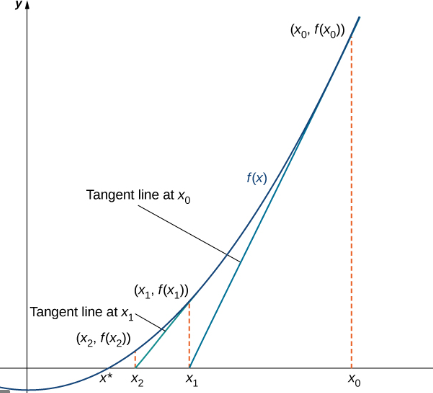# The Newton’s method of obtaining the second approximation by using a graph.### Single Variable Calculus: Concepts...

4th Edition
James Stewart
Publisher: Cengage Learning
ISBN: 9781337687805### Single Variable Calculus: Concepts...

4th Edition
James Stewart
Publisher: Cengage Learning
ISBN: 9781337687805

#### Solutions

Chapter 4, Problem 9RCC

a.

To determine

## The Newton’s method of obtaining the second approximation by using a graph.

Expert Solution

### Explanation of Solution

Given information:

The initial approximation is x1 to a root of the equation f(x)=0 .

Calculations:

Newton’s method of obtaining the second approximation is done by using the formula,

xn+1=xnf(xn)f'(xn)x2=x1f(x1)f'(x1)

For getting the third root the same formula is followed. The process is kept on repeating and it finally converges to a desired root.b.

To determine

Expert Solution

### Explanation of Solution

Given information:

The initial approximation is x1 to a root of the equation f(x)=0 .

Calculations:

Newton’s method of obtaining the second approximation is done by using the formula,

xn+1=xnf(xn)f'(xn)x2=x1f(x1)f'(x1)

For getting the third root the same formula is followed. The process is kept on repeating and it finally converges to a desired root.

c.

To determine

Expert Solution

### Explanation of Solution

Given information:

The initial approximation is x1 to a root of the equation f(x)=0 .

Calculations:

Newton’s method of obtaining the second approximation is done by using the formula,

xn+1=xnf(xn)f'(xn)x2=x1f(x1)f'(x1)

For getting the third root the same formula is followed. The process is kept on repeating and it finally converges to a desired root.

d.

To determine

Expert Solution

### Explanation of Solution

Given information:

The initial approximation is x1 to a root of the equation f(x)=0 .

Calculations:

Newton’s method of obtaining the approximation is done by using the formula,

xn+1=xnf(xn)f'(xn)

Several approximations are taken and the process is kept on repeating until it finally converges to a desired root. Under certain circumstances the method fails or works very slowly. This happens when f'(x1) is close to zero. Also there can be certain situation when the approximation is outside the domain of f . In such cases the method will fail.

### Have a homework question?

Subscribe to bartleby learn! Ask subject matter experts 30 homework questions each month. Plus, you’ll have access to millions of step-by-step textbook answers!